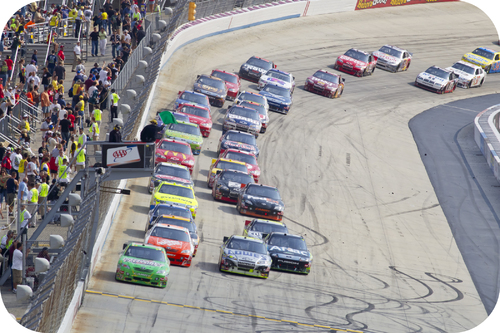LESSON: Distance vs DisplacementIn stockcar races, the winners frequently travel a distance of 500 miles but at the end of the race, their displacement is only a few feet from where they began.

Position, Distance, and Displacement

In order to study how something moves, we must know where it is.  For straight line motion, it is easy to visualize the object on a number line.  The object may be placed at any point on the number line either in the positive numbers or the negative numbers.  It is common to choose the original position of the object to be on the zero mark.  In making the zero mark the reference point, you have chosen a frame of reference.  The position of an object is the separation between the object and the reference point.

When an object moves, we often refer to the amount it moves as the distance.  Distance does not need a reference point and does not need a direction.  If an automobile moves 50 kilometers, the distance traveled is 50 kilometers regardless of the starting point or the direction of movement.  If we wish to find the final position of the automobile, however, just having the distance traveled will not allow us to determine the final position.  In order to find the final position of the object, we need to know the starting point and the direction of the motion.  The change in the position of the object is called its displacement.  The displacement must include a direction because the final position may be either in the positive or negative direction along the number line from the initial position.  The displacement is a vector quantity and vectors are discussed in another section.

Visit the following link for a description of the difference between distance and displacement:

http://www.tutorvista.com/content/physics/physics-i/motion/distance-and-displacement

Example

Problem: An indecisive car goes 120 m North, then 30 m south then 60m North. What is the car's distance and displacement?

Solution:

Distance is the total amount traveled. Thus distance = 120 + 30 + 60 m = 210 m

Displacement is the amount displaced from the starting position. Thus displacement = 120 - 30 + 60 m = 150 m.

Summary

• The length of the actual path traveled by an object is called distance.
• The shortest path between original and final position is called displacement.
• Distance is a scalar and displacement is a vector.
• For motion in a straight line, we can simply add/subtract to find distance and displacement.  For motion in multiple dimensions, we can use Pythagorean Theorem and trigonometry to find displacement.

After you have completed this part of the lesson, you can check the associated box on the main course page to mark it as complete